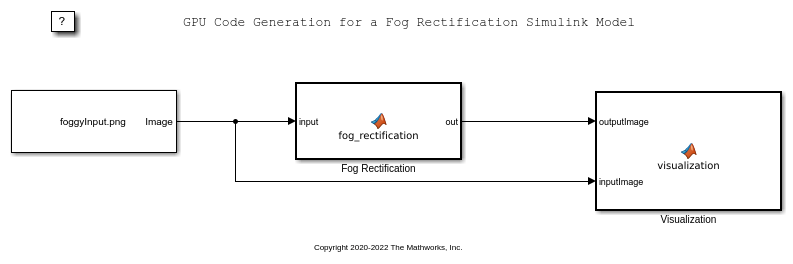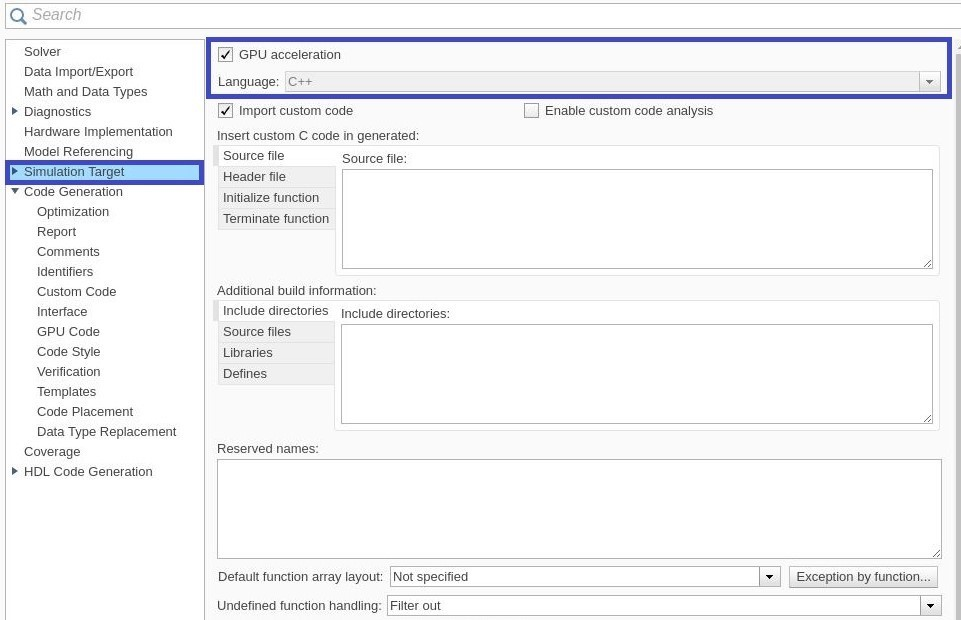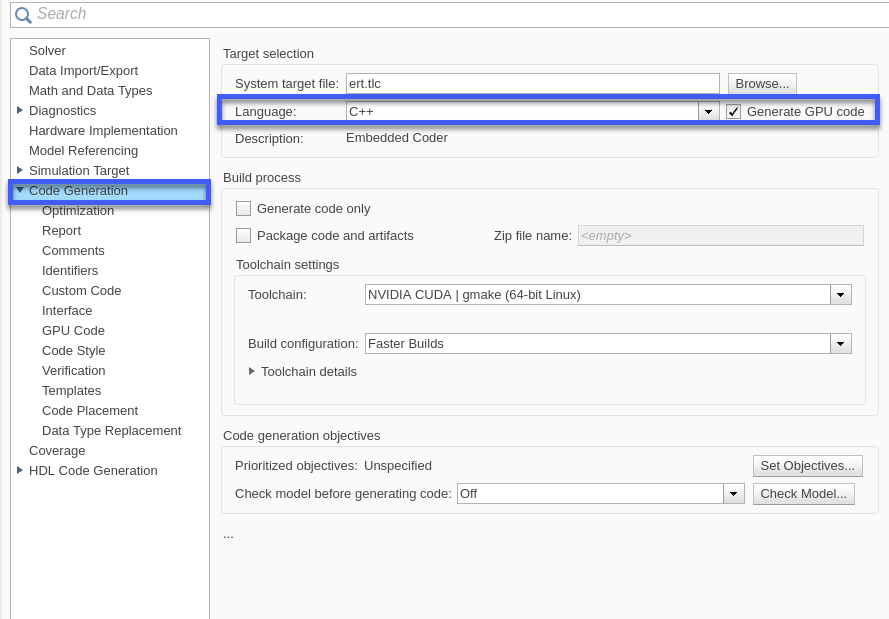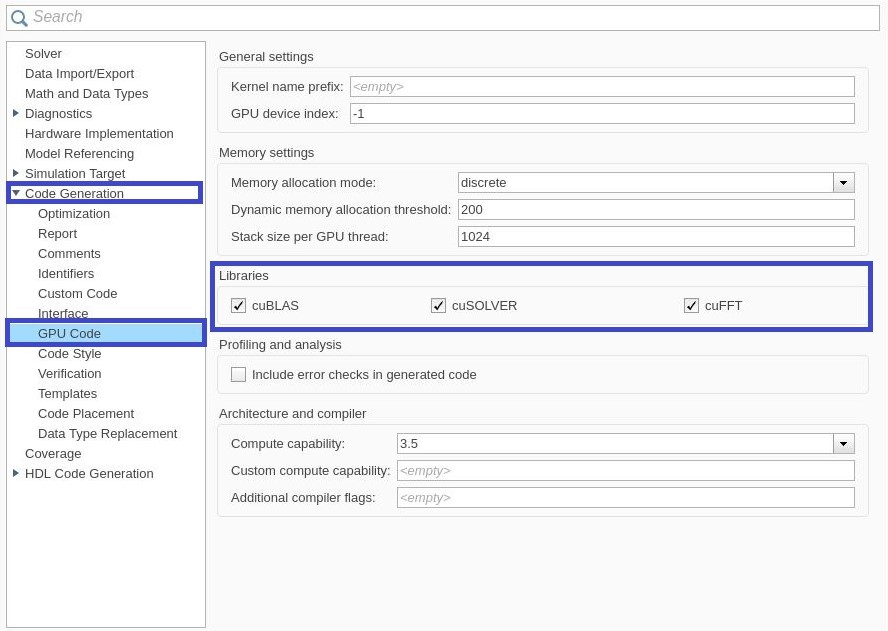# GPU Code Generation for a Fog Rectification Simulink Model

This example demonstrates how to generate CUDA® code from the Simulink® model that takes a foggy image as input and produces a defogged image as output. This example is a typical implementation of fog rectification algorithm. The example uses `conv2`, `rgb2gray`, and `imhist` (Image Processing Toolbox) functions. This example closely follows Fog Rectification example. This example illustrates the following concepts:

• Verification of GPU Environment.

• Model fog rectification application in Simulink by using image processing functions.

• Configure the model for GPU code generation.

• Generate a CUDA executable for the Simulink model.

### Verify GPU Environment

To verify that the compilers and libraries necessary for running this example are set up correctly, use the `coder.checkGpuInstall` function.

```envCfg = coder.gpuEnvConfig('host'); envCfg.BasicCodegen = 1; envCfg.Quiet = 1; coder.checkGpuInstall(envCfg); ```

The Simulink model for fog rectification consists of `Fog Rectification` subsystem that contains a `MATLAB Function` block which takes a foggy image as input and returns a defogged image as output. It uses `fog_rectification` algorithm described in Fog Rectification example. When the model runs, the `Visualization` block displays the foggy input image and defogged output image.

```open_system('fog_rectification_model'); ```### Run model on the Simulation Target

Open Configuration Parameters dialog box.

In Simulation Target pane, select GPU acceleration.Run the Simulation in Normal mode.

```set_param('fog_rectification_model', 'SimulationMode', 'Normal'); sim('fog_rectification_model'); ```### Generate and Build the Simulink Model

In Code Generation pane, select the Language as C++ and enable Generate GPU code.Open Code Generation > GPU Code pane. In the subcategory Libraries, enable cuBLAS, cuSOLVER and cuFFT.Generate and build the Simulink model on the host GPU by using the `rtwbuild` command. The code generator places the files in a build folder, a subfolder named `fog_rectification_model_ert_rtw` under your current working folder.

```status = evalc("rtwbuild('fog_rectification_model')"); ```

### Cleanup

```close_system('fog_rectification_model'); ```